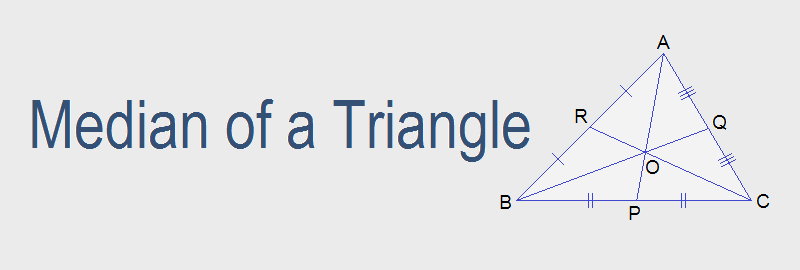# Median of a Triangle## Median of a Triangle

A line segment from a vertex of a triangle to the midpoint of its opposite side is called the median of the triangle. There are three medians of a triangle. Medians of a triangle are concurrent i.e. they passes through a single point which is called the centroid of the triangle.
In the figure AP, BQ and CR are three medians of the triangle ABC. Point O is the centroid of the triangle.

### Properties of medians of a triangle

1.    The median of a triangle divides it into two equal parts. (Median bisects the triangle.)
2.    Three medians of a triangle are concurrent i.e. they passes through a single point which is called centroid of the triangle.
3.   Centroid of a triangle is at a distance two-third of median from the vertex.

### Workout Examples

Example 1: From the given figure, find the area of ΔAMC.

Solution: From the figure,
Area of ΔABC = ½ BC × AD ---------> area of Δ = ½ base × height
= ½ × 6 × 8
= 24 cm2

Area of ΔAMC = ½ of ΔABC ----------> median bisects the triangle
= ½ × 24 cm2
= 12 cm2

Example 2: In the adjoining figure, D is the mid point of AC. If 3DE = BC = 12cm. Find the area of ΔABC.

Solution: Here, 3DE = BC = 12cm
BC = 12cm, and 3DE = 12cm or, DE = 12/3 = 4cm
BD joined.
Area of ΔBCD = ½ BC × DE ---------> area of Δ = ½ base × height
= ½ × 12 × 4
= 24 cm2

Area of ΔABD = Area of ΔBCD ---------> median divides the triangle into two equal parts
= 24 cm2

Area of ΔABC = Area of ΔBCD + ΔABD
= 24 + 24
= 48 cm2

Example 3: In the given figure, D, E, F and H are the mid points of BC, AD, CE and BF respectively. Prove that: ΔEFH = 1/8 ΔABC.

Solution: From the figure,
ΔEFH = ½ of ΔEBF ----------> median EH bisects the ΔEBF
= ½ × ½ of ΔEBC ---------> median BF bisects the ΔEBC
= ½ × ½ × 2ΔEDC ---------> median ED also bisects the ΔEBC
= ½ × ½ × 2 × ½ of ΔADC ---------> median CE bisects the ΔADC
= ½ × ½ × 2 × ½ × ½ of ΔABC ---------> median AD bisects the ΔABC
= 1/8 ΔABC.   Proved.

You can comment your questions or problems regarding the medians of triangle here.